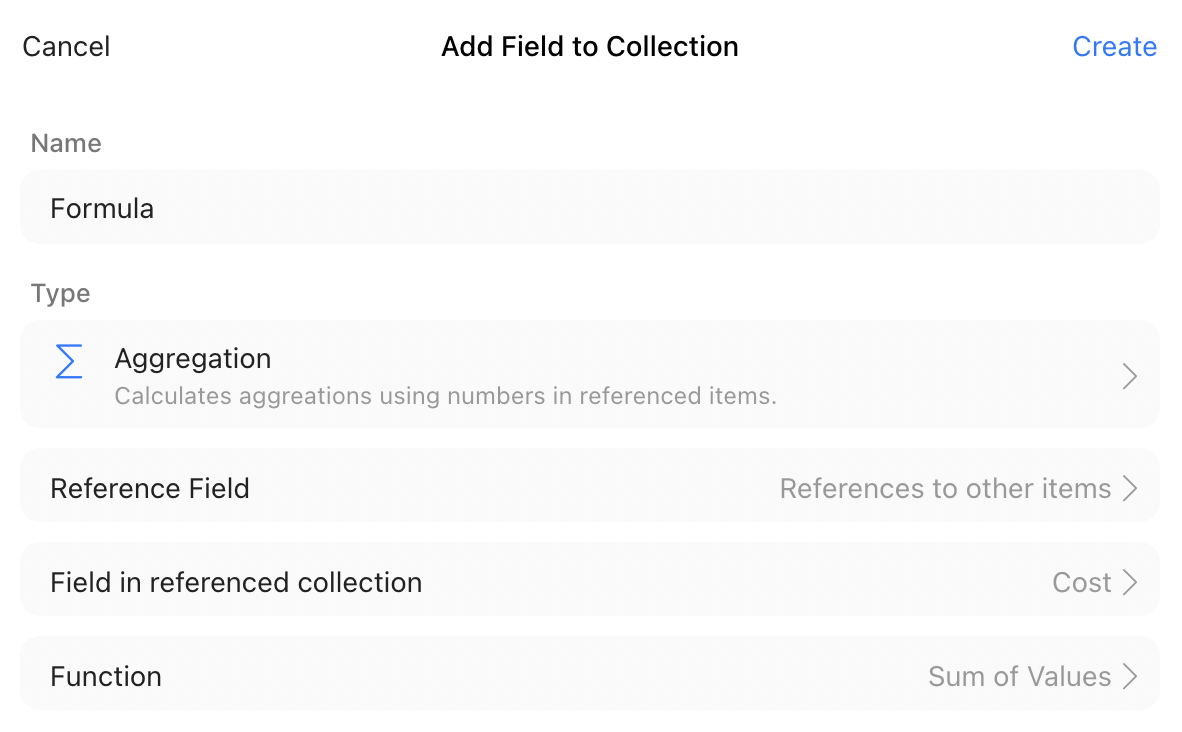Applicable plans
• Free
• Plus
• Enterprise

Formula and aggregation fields allow you to create new information from existing data in the collection, or even from other collections!

When you create a formula field, it applies to every single item in a collection. Think of it as applying a formula to an entire column in a normal spreadsheet. The formula takes in information from other fields in the collection to create new information (in a normal spreadsheet: taking in data from other columns to calculate something for each row).

Formulas

The formula field allows you to create formulas using common operators or functions on number or reference fields in your collection.

For example, if you wanted to calculate the cost of labor for a task, your formula would look like this:

Wage * Time

If you use number fields with spaces in their names, you need to wrap them in braces (Zenkit will do this automatically):

Sum({Material Costs}, {Tool Costs})

Use brackets () as you would in normal mathematical functions to change the order of operations:

({Wage} * {Time}) + Materials

For example, in your spaceship building collection, each task has an ‘Hourly Wage’ field, a ‘Materials Cost’ field, and an ‘Estimated Time’ field. You want to calculate the estimated cost of each task in the project.

You create an ‘Estimated Cost’ formula field that multiplies ‘Hourly Wage’ by ‘Estimated Time’ and adds ‘Material Cost’. The estimated cost for each task is then displayed on each item in the collection!

Guide to using functions and formulas

When you start typing a formula into the formula options field, Zenkit will show you a dropdown of available operators, functions and aggregations you can use.

The first thing you'll be shown is a dropdown list of all number fields in your current collection, or referenced number fields.

Select a field you want to work with from the dropdown menu, or type in a number you want to use.Add an operator then type in another number or choose from the list again.When you're done creating your formula, click 'Create' to apply it to every item in your collection.
When creating formulas, make sure to watch out for braces and brackets! Misplaced brackets can cause your field to throw an error.

Aggregations

Even though you can select it from the 'Add Field' dropdown list, an aggregation will actually create a formula field. An aggregation is essentially a formula-building wizard that makes it easier to build formulas using references.

When you create a new aggregation, it will recognize which reference fields are included in your collection, and pull number fields from inside those references.You then choose the function to apply to those values - a sum, count or average.

Once you click 'Create', Zenkit will create a formula field that sums, counts or averages the number field you chose inside referenced items for each item.

For example, if you have referenced three tasks from your project tasks collection in a main project collection, you can use an aggregation field to sum the cost of those tasks so you can calculate the total sum of the project. You could do the same thing with items from a billing collection linked to a customer in your customers collection, you can create an aggregation that sums the totals of the invoices into a 'Total lifetime value' field. Every time you add a new invoice to that customer, the total lifetime value will update to include it.